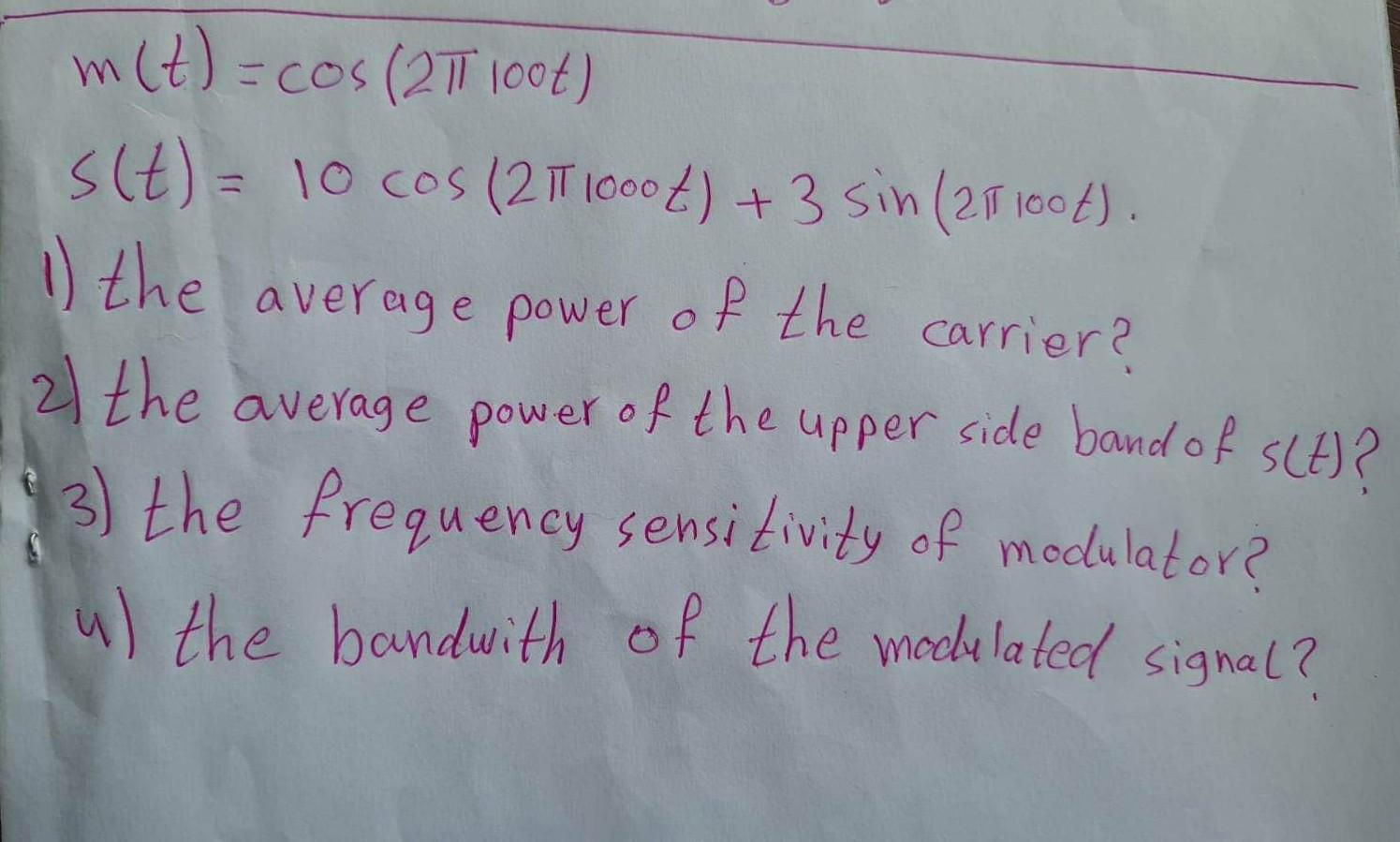Home / Expert Answers / Electrical Engineering / nbsp-begin-array-l-m-t-cos-2-pi-100-t-s-t-10-cos-2-pi-1000-t-3-sin-2-pi-1-pa579

# (Solved):   $\begin{array}{l} m(t)=\cos (2 \pi 100 t) \\ s(t)=10 \cos (2 \pi 1000 t)+3 \sin (2 \pi 1 ...\[ \begin{array}{l} m(t)=\cos (2 \pi 100 t) \\ s(t)=10 \cos (2 \pi 1000 t)+3 \sin (2 \pi 100 t) \end{array}$ 1) the average power of the carrier? 2) the average power of the upper side band of $$s(t)$$ ? 3) the frequency sensitivity of modulator? 4) the bandwith of the modulated signal?

We have an Answer from Expert